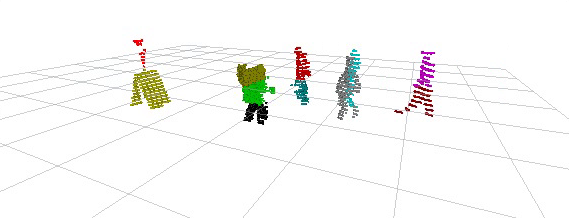# Truncated Bayesian Nonparametrics

Models from Bayesian nonparametrics have infinitely many latent parameters, which has the effect of allowing the number of parameters with an influence on observed data to grow as the amount of observed data increases. In order to exploit conditional independence properties in the model for efficient inference, it is necessary to condition on the latent parameters; but this isn’t possible when there are infinitely many of them. In this work, we address this issue by developing principled finite approximations of priors from Bayesian nonparametrics. These approximations involve truncating a sequential representations of the countable infinity of latent parameters. We develop error bounds in terms of L1 norms on the marginal density of observed data, or alternatively, the probability of the truncated model making a “mistake” when any data point selects a parameter from the truncated tail. This work paves the way for tractable, efficient truncated variational and MCMC inference schemes for nonparametric Bayesian models.### Related Publications

• Campbell, T., Kulis, B., and How, J. P., “Dynamic clustering algorithms via small-variance analysis of Markov chain mixture models,” IEEE Transactions on Pattern Analysis and Machine Intelligence, 2018.